Courses

# Infinite Geometric Progression CA Foundation Notes | EduRev

## CA Foundation : Infinite Geometric Progression CA Foundation Notes | EduRev

The document Infinite Geometric Progression CA Foundation Notes | EduRev is a part of the CA Foundation Course Quantitative Aptitude for CA CPT.
All you need of CA Foundation at this link: CA Foundation

Infinite Geometric Progression

So far, we have found the sum of a finite number of terms of a G. P. We will now learn to find out the sum of infinitely many terms of a G P such as.

1, 1/2, 1/4, 1/8, 1/16, ......

We will proceed as follows: Here a = 1, r = 1/2

The n th term of the G. P. is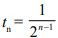, and sum to n terms

i.e;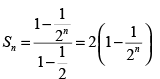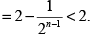So, no matter, how large n may be, the sum of n terms is never more than 2.

So, if we take the sum of all the infinitely many terms, we shall not get more than 2 as answer.

Also note that the recurring decimal 0.3 is really 0.3 + 0.03 + 0.003 + 0.0003 + ... i.e., 0.3 is actually the sum of the above infinite sequence.

On the other hand it is at once obvious that if we sum infinitely many terms of the G. P. 1, 2, 4, 8, 16, ... we shall get a finite sum.

So, sometimes we may be able to add the infinitely many terms of G. P. and sometimes are may not. We shall discuss this question now.

Sum of Infinite Geometric Progression

Let us consider a G. P. with infinite number of terms and common ratio r.

Case 1 : We assume that | r | > 1

The expression for the sum of n terms of the G. P. is then given by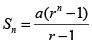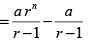......(A)

Now as n becomes larger and larger rn also becomes larger and larger. Thus, when n is infinitely large and | r | > 1 then the sum is also infinitely large which has no importance in Mathematics. We now consider the other possibility.

Case 2 : Let | r | < 1

Formula (A) can be written as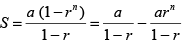Now as n becomes infinitely large, rn becomes infinitely small, i.e., as n → ∞ , rn → 0, then the above expression for sum takes the form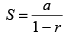Hence, the sum of an infinite G. P. with the first term 'a' and common ratio 'r' is given by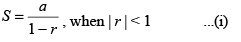Example 1. Find the sum of the infinite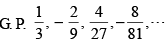Solution :  Here, the first term of the infinite  G. P. is a = 1/3,

and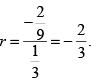Here,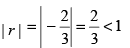∴ Using the formula for sum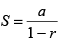we have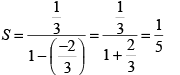Hence, the sum of the given  G. P. is 1/5

Example 2. Express the recurring decimal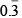as an infinite G. P. and find its value inrational form.

Solution := 0.3333333.....

= 0.3 + 0.03 + 0.003 + 0.0003 + ....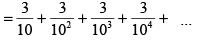The above is an infinite G. P. with the first term a  = 3/10

and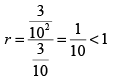Hence, by using the formula, we get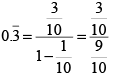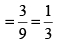Hence, the recurring decimal= 1/3

Example 3. The distance travelled (in cm) by a simple pendulum in consecutive secondsare 16, 12, 9, ... How much distance will it travel before coming to rest ?

Solution : The distance travelled by the pendulum in consecutive seconds are, 16, 12, 9, ... is an infinite geometric progression with the first term a = 16

and r = 12/16 = 3/4 < 1

Hence, using the formula,we get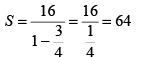∴ Distance travelled by the pendulum is 64 cm

Example 4.   The sum of an infinite G. P. is 3 and sum of its first two terms is 8/3. Find the first term.

Solution : In this problem S = 3. Let a be the first term and r be the common ratio of the given infinite G. P.

Then according to the question.

a + ar = 8/3

or , 3a(1+r) = 8 ......(1)

Hence, using the formula,we get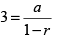or, a = 3 (1 − r)   ......(2)

From (1) and (2), we get.

3.3 (1 – r) (1 + r) = 8

or, 1 - r2 = 8/9

or, r2 = 1/9

or,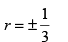from (2)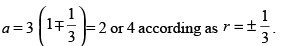Offer running on EduRev: Apply code STAYHOME200 to get INR 200 off on our premium plan EduRev Infinity!

66 docs|68 tests

,

,

,

,

,

,

,

,

,

,

,

,

,

,

,

,

,

,

,

,

,

;